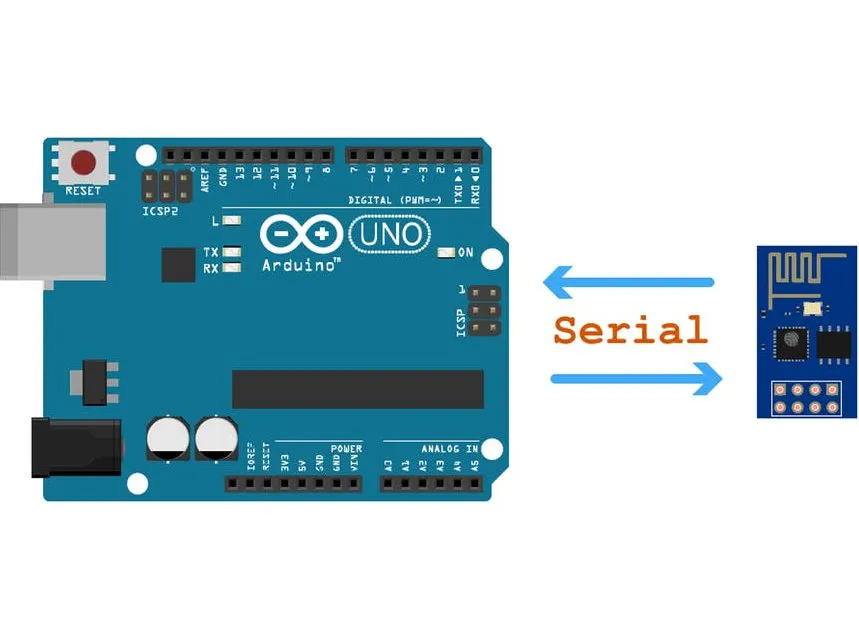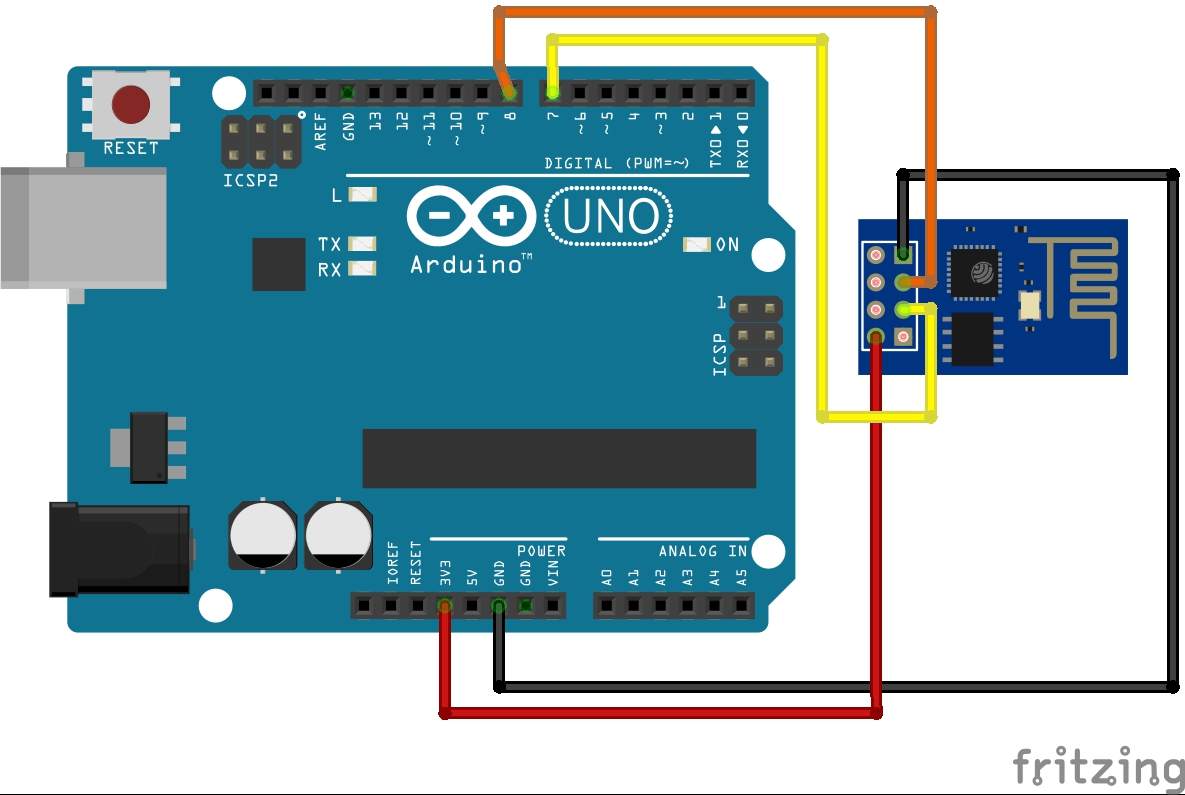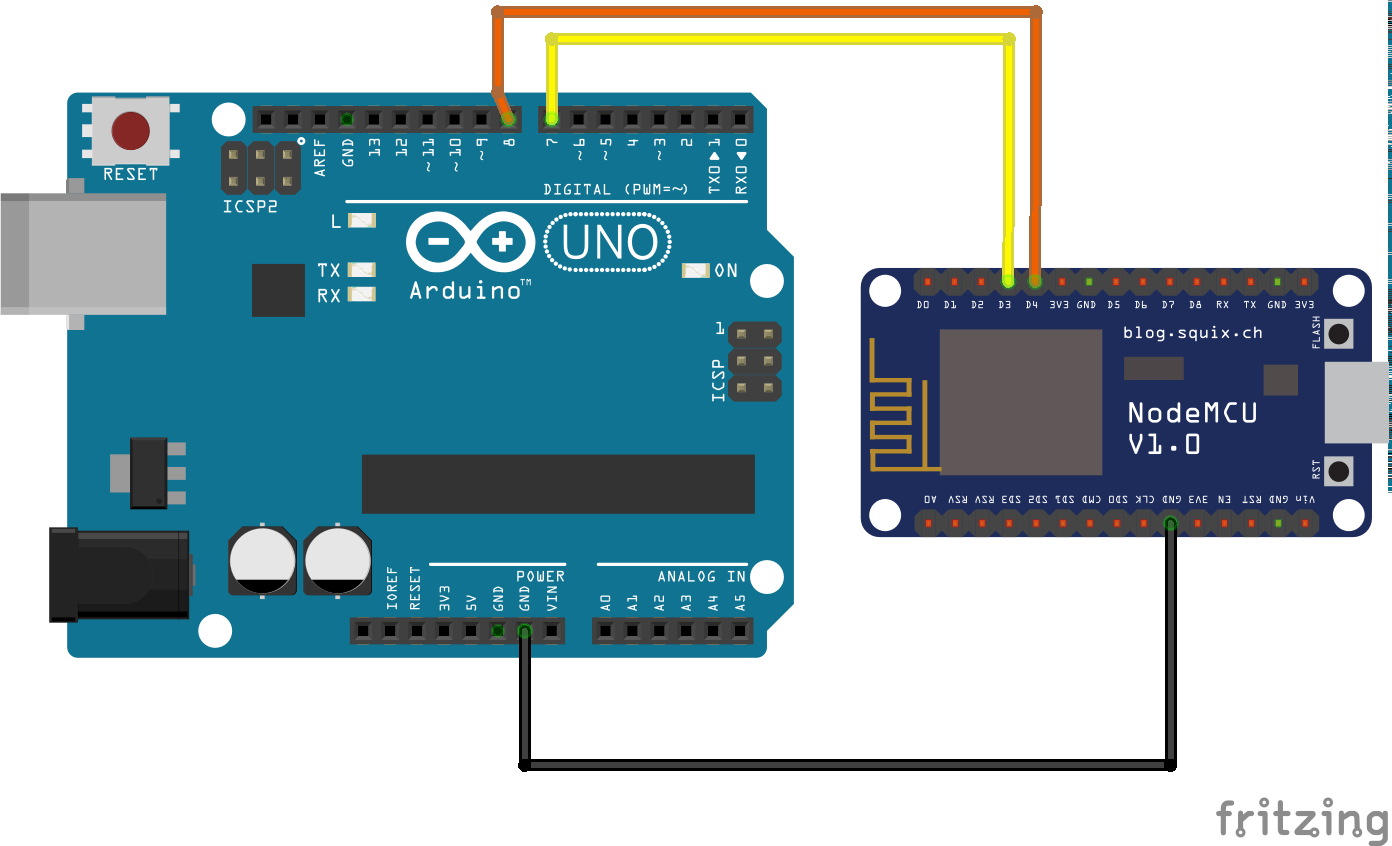Project in progress# Arduino-ESP WiFi Integration © Apache-2.0

Use the program to manage your ESP module with simple commands. This will allow you to access the Internet for any Arduino product.

• 20,170 views
• 62 respects

## Apps and online services

This program solves the ESP module with only simple commands.

• Upload the arduino code to Arduino
• Connect the Arduino digital port 7 with the ESP GPIO0 pin, which is labeled D3!!!
• Connect the Arduino digital port 8 with the ESP GPIO2 pin, which is labeled D4!!!
• If you are not using nodeMCU but ESP-01, you will need a Bi-Directional Logic Level Converter. (this or this)

Then there is nothing more than using the program

``````void wifi(byte id,String data[]){}
void wifi(byte id){}
``````

The wifi function requires a byte data, which is id, or if necessary, additional arguments. To do this, create a String array

``````String arg[]={"Wifi_SSID","WiFi_PASSWD"};
wifi(1,arg);
``````

To extract response data, create a String variable and read op_read() or just print out.

``````String result=op_read();
OR
``````

### Command Codes:

(These are the first parameters of the wifi function)

1 - Connect to wifi. Return: IP address as String

``````String arg[]={"SSID","PASSWD"};
wifi(1,arg);
``````

2 - still empty

3 - scan the available networks. Return: the available netowrks name, Signal Strength, and the security.

``````wifi(3);
``````

4 - Check your Wifi connection Returns: Connected/Disconnected

``````wifi(4);
``````

9 - Disconnect from any networks Return: ok

``````wifi(9);
``````

21-Http client. Return the source code.

``````String arg[]={"http://vanenet.hu/"};
wifi(21,arg);
``````

Principle of operation

I always keep the issued command in the task node named task. The first address is the id that is the ID of the command. (eg 1: connection to the network) and the second address the required arguments divided by "\t". We'll send Serial data to ESP.

The first character is converted to bytes and examined for a task with such an identifier. Then I mapped the inverter of the id, so where there was bit 1 there would be 0 bit and where 0 was 1. (eg, the id of id 1 will be 254) This will be the response identifier. We will execute the command and then add the return value of the function to the answer. We send the response back to the Arduino on the Serial port and check whether the invert value matches the issued command ID. If we return with the result, and if not, then we have already given a new command to the esp, but it has not done the previous one. In this case, read the return values ​​into the wrong variable.

If you are interested in more interesting projects, then follow me and see my previous projects.

## Code

##### arduinoArduino
```#include<SoftwareSerial.h>

SoftwareSerial esp(7, 8);

String wrong;   //Wrong respons buffer

void setup()
{
esp.begin(9600);
Serial.begin(9600);   //start the serial port

String arg[]={"WifiSSID","WIFIPASSWD"}; //create a String array for arguments
wifi(1,arg);    //connect to a network
}

void loop()
{
if (esp.available())
}

{
String buff = esp.readStringUntil(char(244));   //read datas to the "buff" until the character ¶ character (An arbitrary character)
buff.replace(String(char(244)),""); //delet this char from buffer
byte id=byte(buff.charAt(0)); //get the id from the first char of buffer
buff.remove(0,1); //remove the first char. we don't need any more
if ((byte(task.charAt(0)) + id) == 255)  //if the task id and response id are inverts
{
return buff;    //return
}
else
{
Serial.println("Old datas:\n");   //else this are old datas. Read to wrong
wrong=buff.substring(1);
Serial.println(wrong);
}
return "-1";  //this can not happen
}

void wifi(byte id,String data[])  //function wifi with arguments
{
String output=String(char(id)); //add the converted id to output
for(int k=0;k<sizeof(data);k++)   //devide arguments with a "\t" char
output+=data[k]+"\t";
esp.println(output);  //send datas to esp
}
void wifi(byte id)    //This is the same as the previous one, without arguments
{
String output=String(char(id));
esp.println(output);
}
```
##### espArduino
```#include <SoftwareSerial.h>
#include <ESP8266WiFi.h>
#include <ESP8266HTTPClient.h>
#include <WiFiClient.h>

SoftwareSerial ard(0,2);

void setup()
{
WiFi.mode(WIFI_STA); //start wifi as station
Serial.begin(9600);
ard.begin(9600);    //start serial port
}

void loop()
{
if (ard.available())
}

{
task = buff.charAt(0); //set up the id
int ind = 1; //indexing value
for (int l = 0; l < buff.length(); l++)
{
if (buff.charAt(l) == '\t')
ind++;
else
}
byte id = byte(task.charAt(0));    //set up id
byte invert = ~id;    //create invert data
Serial.print("id: "); Serial.print(id); Serial.print("\t"); //print incomeing datas
Serial.print("Invert: "); Serial.println(invert);   //not necessary, just for debuging
String resp = String(char(invert)); //add invert id to response
switch (id)   //check for command
{
case 1:
{
//WiFi client
Serial.print("Resp:\t"); Serial.println(resp.substring(1));
break;
}
case 2:
{
//WiFi AP
break;
}
case 3:
{
//scan network
resp += scan();
Serial.print("Resp:\t"); Serial.println(resp.substring(1));
break;
}
case 4:
{
//is connected?
if (WiFi.status() == WL_CONNECTED)
resp += "connected";
else
resp += "disconnected";
Serial.print("Resp:\t"); Serial.println(resp.substring(1));
break;
}
case 9:
{
WiFi.disconnect();
resp+="ok";
break;
}
case 21:
{
//http client
Serial.print("Resp:\t"); Serial.println(resp.substring(1));
break;
}
}
ard.println(resp);    //send datas
}

{
Serial.println("Wifi connect to");
WiFi.mode(WIFI_STA);  //set up wifi as station
for (int tim = 0; tim < 30; tim++)
{
if (WiFi.status() == WL_CONNECTED)
break;
else
delay(500);
}
//try for 15 seconds connect to network
Serial.println(WiFi.localIP());
if (WiFi.status() == WL_CONNECTED)
return (String(WiFi.localIP()) + "." + String(WiFi.localIP()) + "." + String(WiFi.localIP()) + "." + String(WiFi.localIP()));
else
return "0";
//if we succeed, we will return with the ip address, if we do not return with 0
}

String scan()
{

int n = WiFi.scanNetworks();
if (n == 0)
return "0 networks availabele";
else
{
String ret = "";
for (int i = 0; i < n; ++i) {
ret += WiFi.SSID(i);
ret += "\t";
ret += "\t";
ret += ((WiFi.encryptionType(i) == ENC_TYPE_NONE) ? "Opened" : "Closed");
ret += "\n";
}
return ret;
}
}

String http_client(String URL)
{
if ((WiFi.status() == WL_CONNECTED))
{
WiFiClient client;
HTTPClient http;
if (http.begin(client, URL))
{
int httpCode = http.GET();
if (httpCode > 0)
{
if (httpCode == HTTP_CODE_OK || httpCode == HTTP_CODE_MOVED_PERMANENTLY)
{
http.end();
return payload;   //return with the source code
}
}
else
return "False request";
}
}
else
{
return "Not connected";
}
}
```

## Schematics• 67,926 views
• 47 respects

#### WiFi ESP8266 and DHT22 Sensor

Project tutorial by hbolanos2001

• 37,837 views
• 29 respects

#### AiouLinux

Project showcase by CESARMASSARI

• 7,407 views
• 37 respects

#### Home Automation Using Arduino and WiFi Module ESP01

Project tutorial by Subhajit

• 12,304 views
• 1 comment
• 6 respects

#### WiFi Robot

Project tutorial by Team Kartik

• 6,612 views
• 1 comment
• 16 respects

• 5,646 views
• 1 comment
• 3 respects